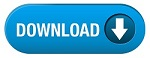Home » SSC » SSC Scientific Assistant Papers : Aptitude

# SSC Scientific Assistant Papers : Aptitude## SSC scientific-assistant papers : Aptitude

1. If the following numbers are rewritten by interchanging the digits in
tens place and hundreds place and then arranging them in the descending order.
What will be the second digit of the newly formed fifth number from your right ?
479, 736, 895, 978, 389, 675

(A) 3
(B) 4
(C) 5
(D) 6

2. P is 60 m South-East of Q. R is 60 m North-East of Q. Then R is in
which direction of P ?

(A) North
(B) North-East
(C) South
(D) South-East

questions that follow On a playing ground A, B, C, D and E are standing as
described below facing the North.

(i) B is 50 metres to the right of D.
(ii) A is 60 metres to the South of B
(iii) C is 40 metres to the West of D.
(iv) E is 80 metres to the North of A.

3. If a boy walks from C, meets D followed by B, A and then E, how many
metres has he walked if he has travelled the straight distance all through ?

(A) 120
(B) 150
(C) 170
(D) 230

4. What is the minimum distance (in metre approximately) between C and E?

(A) 53
(B) 78
(C) 92
(D) 120

5. Who is to the South-East of the person who is to the left of D ?

(A) A
(B) B
(C) C
(D) E

6. A man was walking in the evening just before the sun set. His wife said
that, his shadow fell on his right. If the wife was walking in the opposite
direction of the man, then which direction the wife was facing ?

(A) North
(B) West
(C) South
(D) East

Directions(Q. 7-11) In each of the following questions choose the set of
numbers from the four alternative sets that is similar to the given set.

7. Given set : (4, 9, 18)

(A) (8, 14, 22)
(B) (10, 15, 25)
(C) (6, 12, 23)
(D) (12, 17, 26)

8. Given set : (10, 14, 17)

(A) (4, 11, 14)
(B) (9, 12,
(C) (8, 13, 18)
(D) (6, 9, 12)

9. Given set : (7, 27, 55)

(A) (21, 35 , 52)
(B) (18, 42 , 65)
(C) (16, 40 , 72)
(D) (13, 30 , 58)

10. Given set : (39, 28, 19)

(A) (84, 67 , 52)
(B) (52, 25 , 17)
(C) (70, 49 , 36)
(D) (65, 45 , 21)

## Study Kit for SSC Scientific Assistant (IMD) Examination

11. Given set : (246, 257, 358)

(A) (233, 343, 345)
(B) (273, 365, 367)
(C) (143, 226, 237)
(D) (145, 235, 325)

Directions(Q. 12-16) Each question contains six or seven statements
followed by four sets of combinations of three. Choose the set in which the
statements are logically related.

12.
(1) All books are having pages.
(2) All kings are having pages.
(3) All kings are books.

(B) 4, 2, 6
(C) 1, 5, 3
(D) 2, 4, 5

Directions(Q. 17-21) Each of the questions below consists of a question and
two statements numbered (I) and (II). You have to decide whether the data
provided in the statements are sufficient to answer the question. Give answers

(A) If the data in statement (I) alone are sufficient to answer the question,
while the data in statement (II) alone are not sufficient to answer the
question;
(B) If the data in statement (II) alone are sufficient to answer the question,
while the data in statement (I) alone are not sufficient to answer the
questions;
(C) If the data even in both statements (I) and (II) together are not sufficient
(D) If the data in both statement (I) and (II) together are necessary to answer
the question.

17. In which direction is Mahatmajis statue facing ?

I. The statue is towards the northern end of the city.
II. The statues shadow falls towards East at 5 Oclock in the evening.

18. What is the total number of pupils in the final year class ?

I. The number of boys in the final year class is twice as much as the number
of girls in that class.
II. The sum of the ages of all the pupils in the class is 399 years and their
average age is 19 years.

19. Who is the tallest among A, B, C and D ?

I. A is taller than C.
II. B is taller than C and D.

20. How many Sundays are there in a particular month of a particular year?

I. The month begins on Monday.
II. The month ends on Wednesday.

21. What is the total number of pages in this book ?

I. I counted 132 pages from the beginning of this book.
II. My wife counted 138 pages starting from the end of the same book.

Directions(Q. 22-26) In each of the questions given below, there is a
statement followed by three assumptions numbered I, II and III. An assumption is
something supposed or taken for granted. You have to consider the statement and
assumptions and then decide, which of the assumption(s) is/are implicit in the
statement.

##